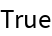#Function Repository Resource:

# SupplementaryAngleDegree

Compute the geometric supplement to a given angle using degrees

Contributed by: Paco Jain (Wolfram Research)
 ResourceFunction["SupplementaryAngleDegree"][θ] gives the supplement to the angle of θ in degrees.

## Details and Options

ResourceFunction["SupplementaryAngleDegree"] takes all angles to be measured in degrees.
The supplement of an angle θ is defined to be the angle that sums with θ to form a straight angle. In other words, 180°-θ.

## Examples

### Basic Examples

Compute the supplement of an angle:

 In:=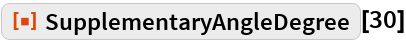Out=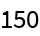### Scope

SupplementaryAngleDegree works directly on Quantity expressions with units of "AngularDegrees":

 In:=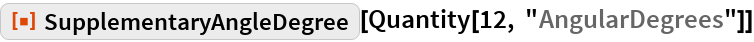Out=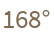SupplementaryAngleDegree works directly on DMS lists of the type returned by DMSList:

 In:=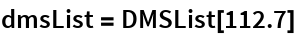Out=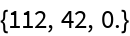In:=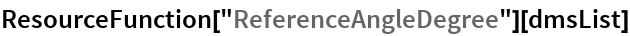Out=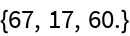SupplementaryAngleDegree works directly on DMS strings of the type returned by DMSString:

 In:=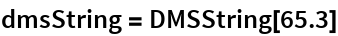Out=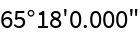In:=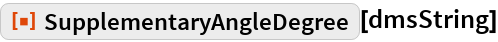Out=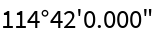### Properties and Relations

SupplementaryAngleDegree will remain unevaluated for non-numeric input:

 In:=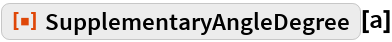Out=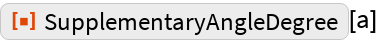SupplementaryAngleDegree will return a negative result if the input angle is greater than 180°:

 In:=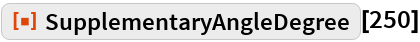Out=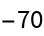For negative input, SupplementaryAngleDegree returns a result greater than 180°:

 In:=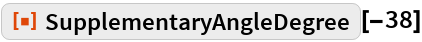Out=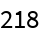For numeric input, SupplementaryAngleDegree is its own inverse:

 In:=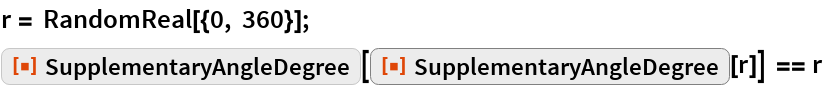Out=